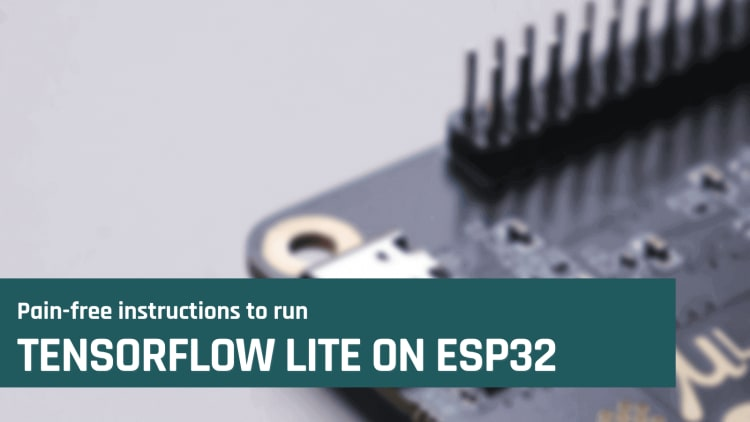# Pain-free instructions to run Tensorflow Lite on ESP32 in Arduino IDE

Convert a TensorFlow Lite model to deploy on your ESP32 board using the Arduino IDERunning TensorFlow Lite on microcontrollers is a pain. No doubt.

If you're just getting started and you follow the official tutorials on the TensorFlow blog or the Arduino website, you'll soon get lost.

At least that's what happened to me.

But it doesn't have to be the same for you. You don't have to go through all the pains I suffered.

Running TensorFlow on your Esp32 board can be as easy as 3 lines of code. You only have to install the `EloquentTinyML` Arduino library and follow the code examples. It works out of the box on ARM and Esp32 chips. No pain, 100% hassle free!

## Create a neural network

For the sake of this tutorial, we'll create a TensorFlow model to predict the sine function. It's a toy model often used to get familiar with TensorFlow.

``````import math
import numpy as np
import tensorflow as tf
from tensorflow.keras import layers
from sklearn.model_selection import train_test_split

def get_model():
x_values = np.random.uniform(low=0, high=2 * math.pi, size=1000)
y_values = np.sin(x_values)
x_train, x_test, y_train, y_test = train_test_split(x_values, y_values, test_size=0.3)
x_train, x_validate, y_train, y_validate = train_test_split(x_train, y_train, test_size=0.3)

# create a NN with 2 layers of 16 neurons
model = tf.keras.Sequential()
model.compile(optimizer='rmsprop', loss='mse', metrics=['mae'])
model.fit(x_train, y_train, epochs=100, batch_size=16, validation_data=(x_validate, y_validate))

return model, x_train, y_train
``````## Export TensorFlow model to Arduino C++

To convert a TensorFlow Lite model into usable Arduino C++ code, we will use a Python package called `everywhereml`. It implements a few methods to convert Machine Learning models to C++.

First of all, install it.

``````pip install everywhereml>=0.2.12
``````

Then, convert the model we created.

``````from everywhereml.code_generators.tensorflow import tf_porter

tf_model, x_train, y_train = get_model()
# tf_porter() requires:
#   1. the neural network model
#   2. the input data (to detect the input dimensions)
#   3. the output labels (to detect the number of classes - if classification)
#
# Passing `instance_name` will create an instance of the model, so you don't have to
# `area_size` is to control how much memory to allocate for the network
# It is a trial-and-error process
porter = tf_porter(tf_model, x_train, y_train)
cpp_code = porter.to_cpp(instance_name='sineNN', arena_size=4096)

print(cpp_code)
``````

Copy the output code and save it to a file, so can be included in your Esp32 Arduino sketch (eg. named `SineNN.h`)

## Run TensorFlow Lite on Esp32 with Arduino IDE

You can run your TensorFlow Lite neural network on the Esp32 with only few lines of code.

You need to install the `EloquentTinyML` library first.

``````You need version >=2.4.4
``````And this is the main Arduino sketch.

``````// replace with your actual file
#include "SineNN.h"

void setup() {
Serial.begin(115200);

while (!sineNN.begin()) {
Serial.print("Error in NN initialization: ");
Serial.println(sineNN.getErrorMessage());
}
}

void loop() {
for (int i = 0; i < 20; i++) {
// pick x from 0 to PI
float x = 3.14f * i / 20.0f;
// even if the input vector is made of a single value
// you ALWAYS need to create an array
float input = { x };

float y_true = sin(x);
// to run the network, call `predict()`
float y_pred = sineNN.predict(input);

Serial.print("sin(");
Serial.print(x);
Serial.print(") = ");
Serial.print(y_true);
Serial.print("\t predicted: ");
Serial.println(y_pred);
delay(1000);
}
}
``````

Two lines are required: `sineNN.begin()` to init the TensorFlow Lite stuff and `sineNN.predict(input)` to get a prediction from the model.

The same exact code works both for Esp32 boards and ARM boards.

You don't have to compile anything on your side, since the library handles all the boring stuff for you.

Pain-free, as I promised!

Now that you know how to run your TensorFlow Lite model on Esp32, you can create your very own project without fear.

If looking for inspiration, you will find a few projects on this website to get started.

## Related posts

libraries

### TinyMLgen for Python

A Python package to export TensorFlow Lite neural networks to C++ for microcontrollers

tinyml

### Arduino WiFi Indoor Positioning

Localize people and objects as they move around your building with the power of Machine Learning

esp32-cam

### Esp32 Camera Object Detection

The beginner-friendly guide to run Edge Impulse FOMO Object Detection on the Esp32 Camera with little effort

tinyml

### Color classification with TinyML

Get familiar with data collection and pre-processing for Machine Learning tasks

libraries

### Eloquent Edge Impulse for Arduino

An Arduino library to make Edge Impulse neural networks more accessible and easy to use